Courses

# Test: Real Numbers - 4

## 25 Questions MCQ Test Mathematics (Maths) Class 10 | Test: Real Numbers - 4

Description
This mock test of Test: Real Numbers - 4 for Class 10 helps you for every Class 10 entrance exam. This contains 25 Multiple Choice Questions for Class 10 Test: Real Numbers - 4 (mcq) to study with solutions a complete question bank. The solved questions answers in this Test: Real Numbers - 4 quiz give you a good mix of easy questions and tough questions. Class 10 students definitely take this Test: Real Numbers - 4 exercise for a better result in the exam. You can find other Test: Real Numbers - 4 extra questions, long questions & short questions for Class 10 on EduRev as well by searching above.
QUESTION: 1

### A number when divided by 61 gives 27 as quotient and 32 as remainder .Find the number

Solution:

Let the number be x
divident = divisor×quotient + remainder
x = 61×27 + 32
= 1679
so, the number will be 1679

QUESTION: 2

Solution:

a = pq2

b = p3q

LCM (a,b) = p3q2

QUESTION: 3

### The HCF and LCM of two numbers is 9 and 459 respectively. If one of the number is 27, then the other number is

Solution:

Using the result, HCF × LCM = Product of two natural numbers ⇒ the other number =  product of two no. = LCM *HCF
Let unknown no. = X
>> X * 27 = 9*459
X = 9*459/27
X = 153

So X=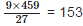So option B is correct answer.

QUESTION: 4

If the HCF of 65 and 117 is expressible in the form 65m – 117, then the value of ‘m’ is

Solution:

First of all find the HCF of 65 and 117,

117 = 1×65 + 52

65 = 1× 52 + 13

52 = 4 ×13 + 0

∴ HCF of 65 and 117 is 13.

65m - 117 = 13

65m = 117+13 = 130

∴m =130/65 = 2

QUESTION: 5

If a is a non-zero rational and √b is irrational, then a√b is:

Solution:

If possible let a√b be rational. Then a√b = p/q,
where p and q are non-zero integers, having no common factor other than 1.
Now, a√b = p/q ⇒ √b = p/aq……….(i)
But, p and aq are both rational and aq ≠ 0.
∵ p/aq is rational.
Therefore, from eq. (i), it follows that √b is rational.
The contradiction arises by assuming that a√b is rational.
Hence, a√b is irrational.

QUESTION: 6

The decimal expansion of number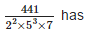Solution:

A number with terminal decimal expansions have the denominator  in the form,
2m 5where m & n ∈ W.
The number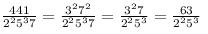Which the denominator is in the form,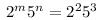with m = 2 , n = 3.
Hence, it has terminal decimal expansion.

QUESTION: 7

Every positive even integer is of the form ____ for some integer ‘q’.

Solution:

Let a be any positive integer and b = 2
Then by applying Euclid’s Division
Lemma, we have, a = 2q + r where 0 ⩽ r < 2 r = 0 or 1
Therefore, a = 2q or 2q+1
Therefore, it is clear that a = 2q i.e.,
a is an even integer in the form of 2q

QUESTION: 8

The HCF of 867 and 255 is

Solution:

As, 867=255 × 3 +102
255 = 102 × 2 + 51
102 = 51 × 2 + 0
So, HCF (867,255) = 51

QUESTION: 9

If HCF(a, b) = 12 and a × b = 1800, then LCM(a, b) is

Solution:

Using the result, HCF × LCM = Product of two natural numbers ⇒ LCM (a, b) = 1800/12 = 150

QUESTION: 10

If d is the HCF of 56 and 72, then values of x,y satisfying d = 56 x+72y :

Solution:

Since, HCF of 56 and 72, by Euclid’s divsion lemma,
72 = 56 × 1 + 16 ……….(i)
56 = 16 ×3 + 8 ……….(ii)
16 = 8× 2 + 0 ……….(iii)
∴ HCF of 56 and 72 is 8.
∴ 8 = 56 – 16× 3
8 = 56 – (72 – 56 ×1) ×3
[From eq. (i) : 16 = 72 – 56× 1]
8 = 56 – 3 ×72 + 56× 3
8 = 56 × 4 + (–3) × 72
∴ x = 4,y = −3

QUESTION: 11

The number (√3+√5)2 is

Solution: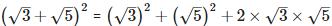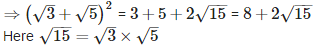Since √3 and √5 both are irrational number therefore (√3+√5)2 is an irrational number.

QUESTION: 12

The largest number which divides 70 and 125, leaving remainders 5 and 8 respectively, is

Solution:

Since, 5 and 8 are the remainders of 70 and 125, respectively. Thus, after subtracting these remainders from the numbers, we have the numbers 65 = (70-5),
117 = (125 – 8), which is divisible by the required number.
Now, required number = HCF of 65,117                                     [for the largest number]
For this, 117 = 65 × 1 + 52 [∵ dividend = divisior × quotient + remainder]
⇒ 65 = 52 × 1 + 13
⇒ 52 = 13 × 4 + 0
∴ HCF = 13
Hence, 13 is the largest number which divides 70 and 125, leaving remainders 5 and 8.

QUESTION: 13

Every positive odd integer is of the form ________ where ‘q’ is some integer.

Solution:

Let a be any positive integer and b = 2.
Then by applying Euclid’s Division
Lemma,
we have, a = 2q+r
where 0 ⩽ r < 2 ⇒ r = 0 or 1 ∴ a = 2q or 2q+1
Therefore, it is clear that a = 2q i.e., a is an even integer.
Also 2q and 2q+1 are two consecutive integers, therefore, 2q+1 is an odd integer.

QUESTION: 14

The LCM of 23×32 and 22×33 is

Solution:

L.C.M. of 23×33 and 22×32 is the product of all prime numbers with greatest power of every given number = 23×33

QUESTION: 15

The HCF of the smallest prime number and the smallest composite number is

Solution:

Smallest prime number = 2 and smallest composite number = 4
∴ HCF (2, 4) = 2

QUESTION: 16

Which of the following is false:

Solution:

H.C.F.(p,q,r)× L.C.M.(p,q,r) ≠ p×q×r. This condition is only applied on HCF and LCM of two numbers.

QUESTION: 17

The number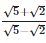is

Solution: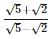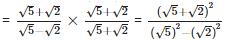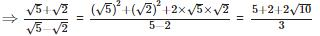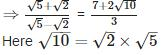Since √2 and √5 both are irrational number thereforeis an irrational number.

QUESTION: 18

The decimal expansion of 987/10500  will terminate after

Solution: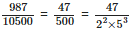Here, in the denominator of the given fraction the highest power of prime factor 5 is 3, therefore, the decimal expansion of the rational number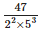will terminate after 3 decimal places.

QUESTION: 19

For any two positive integers a and b, there exist (unique) whole numbers q and r such that

Solution:

Euclid’s Division Lemma states that for given positive integer a and b, there exist unique integers q and r satisfying a = bq+r ; 0 ⩽ r < b.

QUESTION: 20

The least positive integer divisible by 20 and 24 is

Solution:

Least positive integer divisible by 20 and 24 is LCM (20, 24). 20
= 22×5 24=23×3
∴ LCM (20, 24) = 23x 3 x 5 = 120

QUESTION: 21

The largest number which divides 245 and 1029 leaving remainder 5 in each case is

Solution:

When 245 and 1029 are divided by the required number then there is left 5 as remainder. It means that 245 - 5 = 240 and 1029 - 5 = 1024 will be completely divisible by the required number.
Now we determine the HCF of 240 and 1024 by Euclid's Algorithm.
1024 = 240 x 4 + 64
240 = 64 x 3 + 48
64 = 48 x 1 + 16
48 = 16 x 3 + 0
Since remainder comes zero with last divisor 16,
required number = 16

QUESTION: 22

What is the number x? The LCM of x and 18 is 36. The HCF of x and 18 is 2.

Solution:

LCM x HCF = First number x Second number
∴ Required number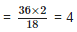QUESTION: 23

If ‘a’ and ‘b’ are both positive rational numbers, then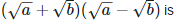Solution: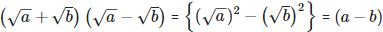= (a−b)
Since a and b both are positive rational numbers, therefore difference of two positive rational numbers is also rational.

QUESTION: 24

The decimal expansion of 21/24 will terminate after

Solution: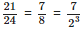Here, in the denominator of the given fraction the highest power of prime factor 2 is 3, therefore, the decimal expansion of the rational number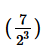will terminate after 3 decimal places.

QUESTION: 25

If the HCF of 65 and 117 is expressible in the form 65m – 117, then the value of m is

Solution:

Find the HCF of 65 and 117,

117 = 1×65 + 52

65 = 1× 52 + 13

52 = 4 ×13 + 0

∴ HCF of 65 and 117 is 13.

65m - 117 = 13

65m = 117+13 = 130

∴m =130/65 = 2